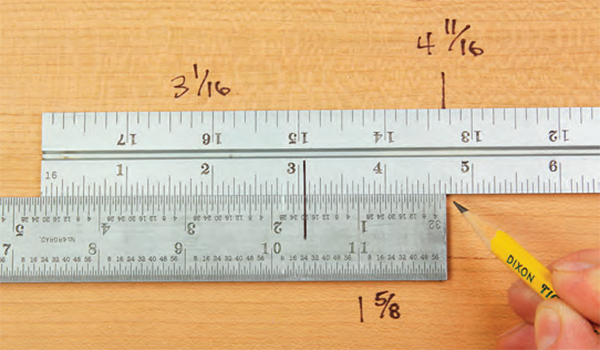# An Easy Way to Tackle FractionsHere’s an old carpenter’s trick for solving fractions that works either with two tape measures or steel rules. If you need to add or subtract two fractional measurements, place the two side by side and align them according to the lengths that need to be added or subtracted. It doesn’t matter if the denominators of the fractions match, because you’re simply comparing two distances, not computing two fractions. For instance, in the photo here, 3-1⁄16″ (top rule) + 1-5⁄8″ (bottom rule) = 4-11⁄16″. The ends of their overlaps tell you the sum. Subtract the same way: the end of the overlap minus one rule’s length shows you the answer. No complicated math required.

-Charles Mak
Calgary, Alberta